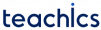# Arithmetic Expressions and Operator Precedence in C

An arithmetic expression is a combination of variables, constants, and arithmetic operators. Arithmetic operators supported in C are +, -, *, / and %. The operands include integer and floating-type numbers. Some algebraic expressions and their corresponding C expressions are given in the following table.

Algebraic Expression   C Expression(a + b) * (a-b)(a * b) / c2 * x * x + 3 * x

Arithmetic expressions are evaluated using an assignment statement of the form variable = expression. The expression is evaluated first and the value is assigned to the variable. An example of an evaluation statement is, c = a - b / d + e

## Precedence of Arithmetic Operators in C

To determine the meaning and value of an expression in an unambiguous manner, we apply the operator precedence and associativity rules. Arithmetic expressions without parentheses are evaluated from left to right using the rules of operator precedence. In C, arithmetic operators have two different priority levels.

• Hight priority * / %
• Low priority + -

The basic procedure for evaluating an expression includes two passes from left to right. The high priority operators are applied during the first pass and the low priority operators are applied in the second pass.

For example, the statement x = 8 – 15 / 5 + 2 * 5 – 7 is evaluated as follows,

• First pass
• x = 8 - 5 + 2 * 5 -7
• x = 8 - 5 + 10 - 7
• Second pass
• x = 3 + 10 - 7
• x = 13 - 7
• x = 6

These steps are illustrated in the following figure.

However, parentheses can be used to change the order in which an expression is evaluated. The expression within parentheses assumes the highest priority. The following rules are used for evaluating expressions containing parentheses.

• Parenthesized subexpressions are evaluated from left to right.
• When there are nested parentheses, the evaluation starts with the innermost sub-expression.
• The order of application of operators in evaluating sub-expressions is determined using the precedence rule.
• When two or more operators with the same precedence level exist in a sub-expression, the associativity rule is applied.
• Arithmetic expressions are evaluated from left to right using the rules of precedence.
• The expressions within parentheses assume the highest priority.

For example, the statement x = 8 – 14 / (5 + 2) * (8 – 7) is evaluated as follows,

• First pass
• x = 8 - 14 / 7 * (8 -7)
• x = 8 - 14 / 7 * 1
• Second pass
• x = 8 - 2 * 1
• x = 8 - 2
• Third pass
• x = 6

## Operator Precedence and Associativity in C

The following table shows the complete list of C operators, their precedence levels, and their rules of association.

Operator Description Associativity Rank
() Function call Left to right 1
[] Array element reference
+ Unary plus Right to left 2
Unary minus
++ Increment
Decrement
! Logical negation
~ Ones complement
* Pointer reference
sizeof Size of an object
(type) Type cast (conversion)
* Multiplication Left to right 3
/ Division
% Modulus
+ Addition Left to right 4
Subtraction
<< Left shift Left to right 5
>> Right shift
< Less than Left to right 6
<= Less than or equal to
> Greater than
>= Greater than or equal to
== Equality Left to right 7
!= Inequality
& Bitwise AND Left to right 8
^ Bitwise XOR Left to right 9
| Bitwise OR Left to right 10
&& Logical AND Left to right 11
|| Logical OR Left to right 12
?: Conditional operator Right to left 13
= Assignment operator Right to left 14
, Comma operator Left to right 15

Subscribe
Notify of